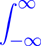EqWorld The World of Mathematical EquationsExact Solutions > Integral Equations > Fredholm Integral Equations of the Second Kind and Related Linear Integral Equations with Constant Limits of Integration## 4. Fredholm Integral Equations of the Second Kind

### 4-4. Integral equations with kernels involving arbitrary functions

1. y(x) −K(x − t) y(t) dt = f(x).
2. y(x) −K(x − t) y(t) dt = f(x).    Wiener--Hopf equation of the second kind.

The EqWorld website presents extensive information on solutions to various classes of ordinary differential equations, partial differential equations, integral equations, functional equations, and other mathematical equations.